# Samacheer Kalvi 6th Maths Solutions Term 3 Chapter 2 Integers Additional Questions## Tamilnadu Samacheer Kalvi 6th Maths Solutions Term 3 Chapter 2 Integers Additional Questions

Question 1.
Write all the integers between – 5 and 10.
Solution:
On the number line, the number increases as we move to right and decreases as we move to the left
∴ -4, -3, -2, -1, 0, 1, 2, 3, 4, 5, 6, 7, 8, 9.

Question 2.
Write the opposite of each of the following
a) Profit of ₹ 500
b) Deposit of ₹ 2000
c) 50 km towards south
Solution:
The opposite of each are
a) loss of ₹ 500
b) withdrawal of ₹ 2000
c) 50 km towards North.

Question 3.
Write the succeeding number of the following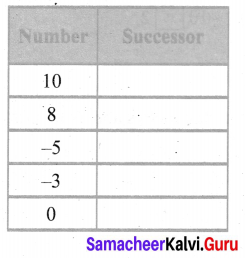Solution:
We know that one more than the given number is its successor.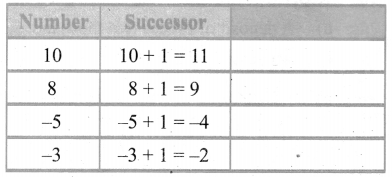Question 4.
Write the preceding number of the following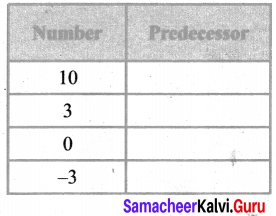Solution:
We know that one more than the given number is its successor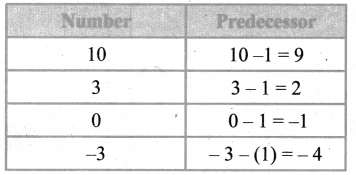Question 5.
Write the following numbers with appropriate signs.
a) 100 m below sea level
b) 25°C above 0°C
c) Any 3 numbers less than 0
Solution:
a) -100 m
b) + 25°C
c) -1, -2, -3

Question 6.
Mark -4, -6, -2 and 3 on the number line
Solution: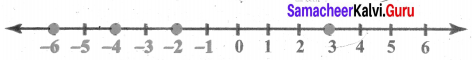Question 7.
Draw a number line and answer the following.
a) Which number will we reach, if we move 4 numbers to the right of-2?
b) Which number will we reach, if we move 5 numbers to the left of 1?
c) If we are at -8 on the number line, in which direction should we move to reach -13?
d) If we are at -6 on the number line in which direction should we move to reach -1 ?
Solution: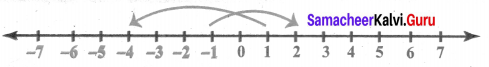(a) 2
(b) -4
(c) left
(d) right

Question 8.
a) Write four negative integers greater than -15?
b) Write four negative integers less than -5?
Solution:
a) -11, -10, -5, -1,
b) -7, -15, -18, -20.Question 9.
Compare the following using < or >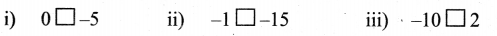Solution: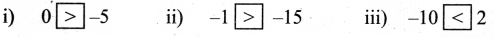Question 10.
Represent the following as integers
a) A deposit of rupees two thousand
b) An aeroplane is flying at a height of three thousand metres above the ground.
Solution:
a) + ₹ 2000
b) + 3000 m